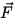# Work

Definition: The work done by an agent exerting a constant force () and causing a displacement () equals the magnitude of the displacement, s, times the component ofalong the direction of. In Figure 5.1, the work done byis:
 W = s Fcos.Note:

• If= 0W = 0. (ie: no work is done when holding a heavy box, or pushing against a wall).
• W = 0 if(ie: no work is done by carrying a bucket of water horizontally).
• The sign of W depends on the direction ofrelative to: W > 0 when component ofalongis in the same direction as, and W < 0 when it is in the opposite direction. This sign is given automatically if we writeas the angle betweenandand write W = Fscos.
• Ifacts along the direction ofthen W = Fs , since cos= cos 0 = 1.
• Work is a scalar.
• The SI units of work are Joules (J) (1 Joule = 1 Newtonmeter). In cgs units: 1 erg = 1 dynecm.Next: Kinetic Energy and the Up: Work and Energy Previous: Work and Energy